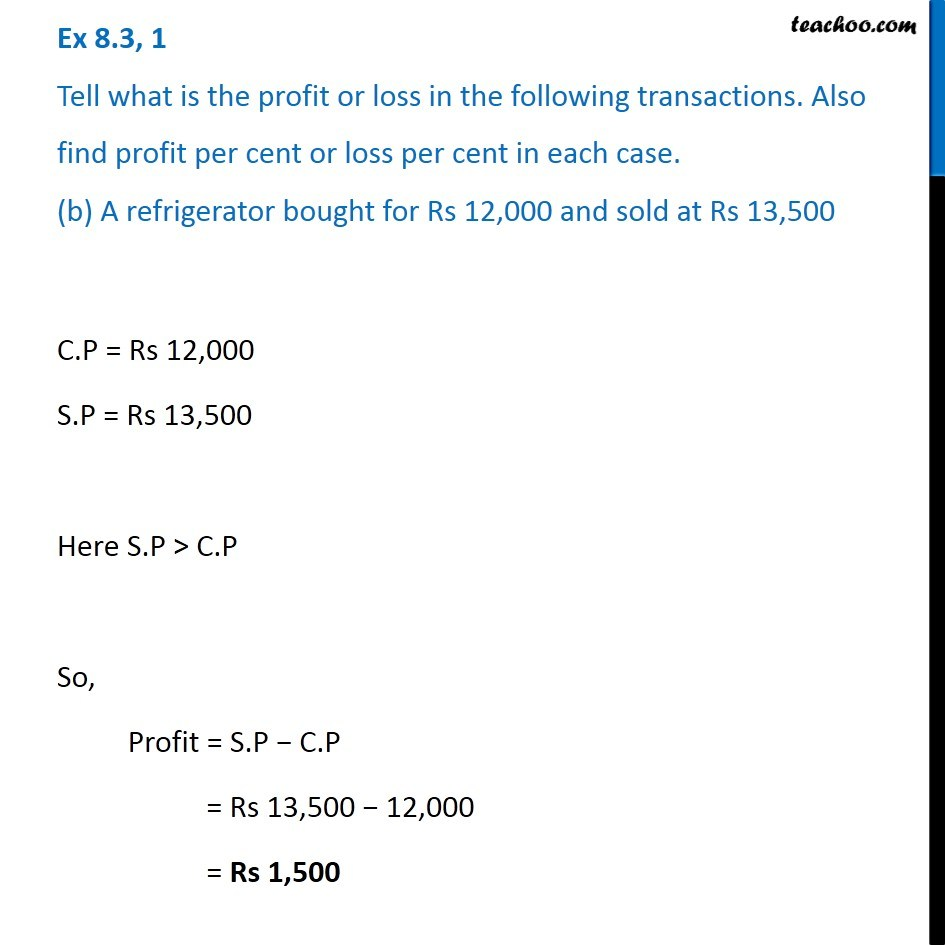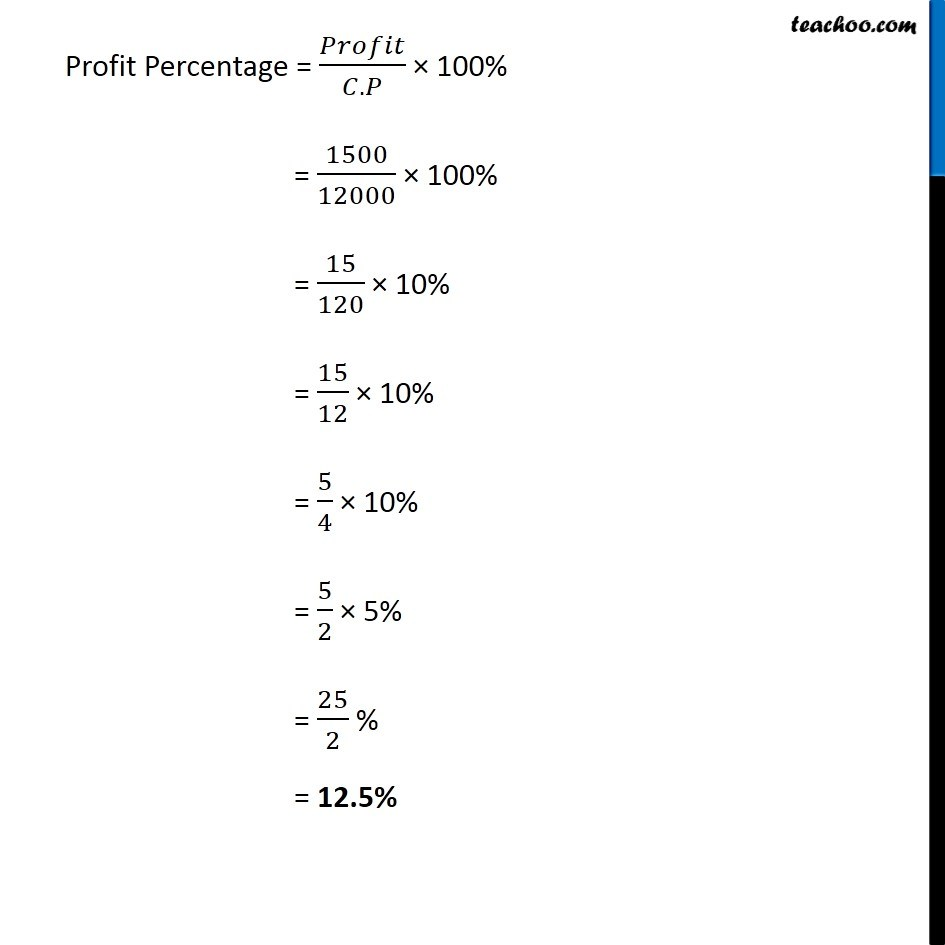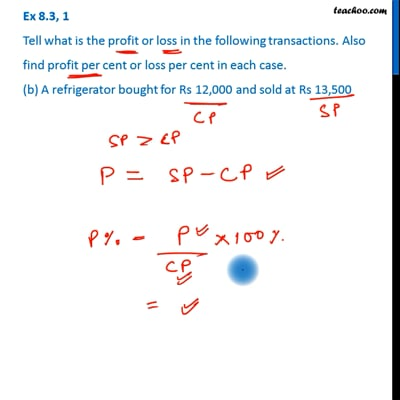Ex 8.3

Chapter 8 Class 7 Comparing Quantities
Serial order wiseThis video is only available for Teachoo black users

Get live Maths 1-on-1 Classs - Class 6 to 12

### Transcript

Ex 8.3, 1 Tell what is the profit or loss in the following transactions. Also find profit per cent or loss per cent in each case. (b) A refrigerator bought for Rs 12,000 and sold at Rs 13,500 C.P = Rs 12,000 S.P = Rs 13,500 Here S.P > C.P So, Profit = S.P − C.P = Rs 13,500 − 12,000 = Rs 1,500 Profit Percentage = 𝑃𝑟𝑜𝑓𝑖𝑡/(𝐶.𝑃) × 100% = 1500/12000 × 100% = 15/120 × 10% = 15/12 × 10% = 5/4 × 10% = 5/2 × 5% = 25/2 % = 12.5%